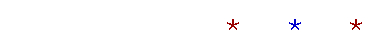# PRECALCULUS

Home

What is a rational number? Which numbers have rational square roots?  The decimal representation of irrationals. What is a real number?13.  Functions

What is a function? The domain and the range.
Functional notation. The argument.
A function of a function.

The graph of a function. Coördinate pairs of a function. The height of the curve at x.

15.  Basic graphs

The constant function. The identity function.
The absolute value function. The parabola.
The square root function. The cubic function.
The reciprocal function.

Variables versus constants.
Definition of a polynomial in x.
The degree of a term and of a polynomial.
The general form of a polynomial.
Domain and range.

A polynomial equation. The roots of a polynomial.
The x- and y-intercepts of a graph.
The relationship between the roots and the x-intercepts.

Definition of the slope. Positive and negative slope. A straight line has only one slope.
"Same slope" and "parallel." Perpendicular lines.
The slope and one point specify a straight line.

The equation of the first degree. The graph of a first degree equation: a straight line.
The slope-intercept form, and its proof.

The sum and product of the roots.

The remainder theorem.

The factor theorem. The fundamental theorem of algebra. The integer root theorem. Conjugate pairs.

Concave upward, concave downward.

Reflection about the x-axis. Reflection about the y-axis. Reflection through the origin.

Symmetry with respect to the y-axis. Symmetry with respect to the origin. Test for symmetry.
Odd and even functions.

Definition of a translation.
The equation of a circle.
The vertex of a parabola.
Vertical stretches and shrinks.

Singularities. The reciprocal function.
Horizontal and vertical asymptotes.Definition of inverses. Constructing the inverse.
The graph of an inverse function.

20.  Logarithms

The system of common logarithms.
The system of natural logarithms.
The three laws of logarithms.
Change of base.

Inverse relations.
Exponential and logarithmic equations.
Creating one logarithm from a sum.23.  Factorials

The Fundamental Principle of Counting.
Factorial representations.
A binomial distribution.

Pascal's triangle.

A proof of the binomial theorem.Home